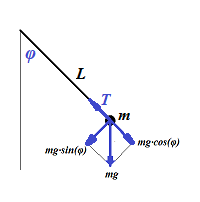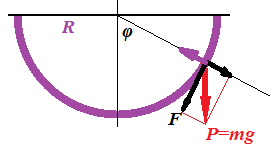## Saturday, October 30, 2021

### Problems 1: UNIZOR.COM - Physics4Teens - Waves - Mechanical Oscillations

Notes to a video lecture on http://www.unizor.com

Problems 1

Problem A

Analyze the oscillations of a pendulum in a uniform gravitational field.Solution

The picture above defines a pendulum with a thread length L and a point-mass m hanging in a gravitational field that produces a constant gravity acceleration g.

Let angle φ be a deviation of the thread from a vertical. This angle is a function of time, so we will use notation φ(t) and will attempt to include it in some equation in order to find this function.

There are two forces acting on a point-mass m: gravity, directed vertically down and equal to m·g, and tension of a thread T.
The force of gravity can be represented as a sum of two forces: directed along a thread and equal to m·g·cos(φ(t)) and perpendicular to a thread equal to m·g·sin(φ(t)).

The tension force T balances the force m·g·cos(φ(t)), as both directed along a thread in opposite directions, thus canceling each other.
The force m·g·sin(φ(t)) is the source of pendulum moving back an force, as it is always directed towards a vertical position of equilibrium.

For the purposes of this analysis we assume that initially we have moved a pendulum from its initial position, so its thread makes an angle φ(0)=φ0 with a vertical and let it go without any push, so φ'(0)=0.

According to the Second Newton's Law, the force is equal to a product of the mass and its linear acceleration.
Therefore, the force F=m·g·sin(φ(t)) that always acts along a tangential to a trajectory of the point-mass towards its equilibrium point should be equal by absolute value to a product of mass m and linear acceleration along a circular trajectory on a thread of the length L, which is equal to L·φ"(t).

Also, this force F always acts towards the vertical. Choose a positive direction of the deviation φ from a vertical as counterclockwise. For positive angles φ, as on the picture above, the direction of the force F would be negative. For negative angles φ the direction of the force F would be positive.
Therefore, the signs of the force F and deviation from the vertical are opposite to each other. For small angles φ the sign of sin(φ) would be the same as the sign of φ.

It results in the following differential equation
−m·g·sin(φ(t)) = m·L·φ"(t)
or
φ"(t) + (g/L)·sin(φ(t)) = 0

Since mass m can be canceled out, our first very important conclusion is that pendulum oscillation does not depend on the mass of an object hanging at its end.

The differential equation above is quite complex and we will not attempt to solve it exactly. However, as many physicists do, we can approximate it under certain conditions.
The condition usually accepted is that the angle of deviation φ(t) is very small and, consequently, function sin (φ(t)) is almost identical to function φ(t).

That brings us to an equation
φ"(t) + (g/L)·φ(t) = 0
which is easy to solve, as it is a regular equation for harmonic oscillations.
The general solution to it, as explained in the previous lecture, is
φ(t) = C1·cos(ωt) + C2·sin(ωt)
where ω=√g/L.
Constants C1 and C2 are determined by initial conditions.

In case of initial conditions φ(0)=φ0 and φ'(0)=0 the values of these constants are:
C1 = φ0
C2 = 0

The solution of pendulum oscillations in this case is
φ(t) = φ0·cos(√g/L·t)
The angular frequency of these oscillations is
ω=√g/L.
The period of oscillations is
T = 2π/ω = 2π√L/g.
The amplitude is
A = φ0.

Just as a reminder, this analysis is applicable only to small oscillations of a pendulum around its equilibrium point, when an angle (in radians) and its sine are approximately equal to each other. Obviously, for any degree of precision the term "small" has its own meaning, the greater the precision - the smaller angle our analysis is applicable to.

Problem B

Analyze the oscillations of a ball of mass m rolling inside a semicircle of radius R in the uniform gravitational field with gravity acceleration g.Solution

The diagram above depicts the forces acting on a ball rolling inside a semicircle.

The weight P=m·g can be represented as a sum of tangential force F=P·sin(φ(t)) and normal to a circle force that is canceled by a reaction of a circle surface.
We should take into account that the direction of force F is always opposite in sign to a sign of angle φ(t). So, the correct expressions for force F is
F = −m·g·sin(φ(t))
According to Newton's Second Law, the force F can be equated to the product of mass and linear acceleration R·φ"(t) of the rolling ball.
F = m·R·φ"(t)
This can be expressed as a differential equation for an angle of position φ(t).
−m·g·sin(φ(t)) = m·R·φ"(t) or, canceling mass m and dividing by radius R,
φ"(t) + (g/R)·sin(φ(t)) = 0

As you see, the result is equivalent to the one we have obtained for a pendulum in the previous problem.

This differential equation is not easily solvable, so we will do exactly as in the previous problem - assume that an angle φ is so small that sin(φ(t)) can be approximated with φ(t), which leads us to a familiar differential equation for harmonic oscillations
φ"(t) + (g/R)·φ(t) = 0

General and specific solutions of this equation are exactly as in the case of a pendulum in Problem A above.